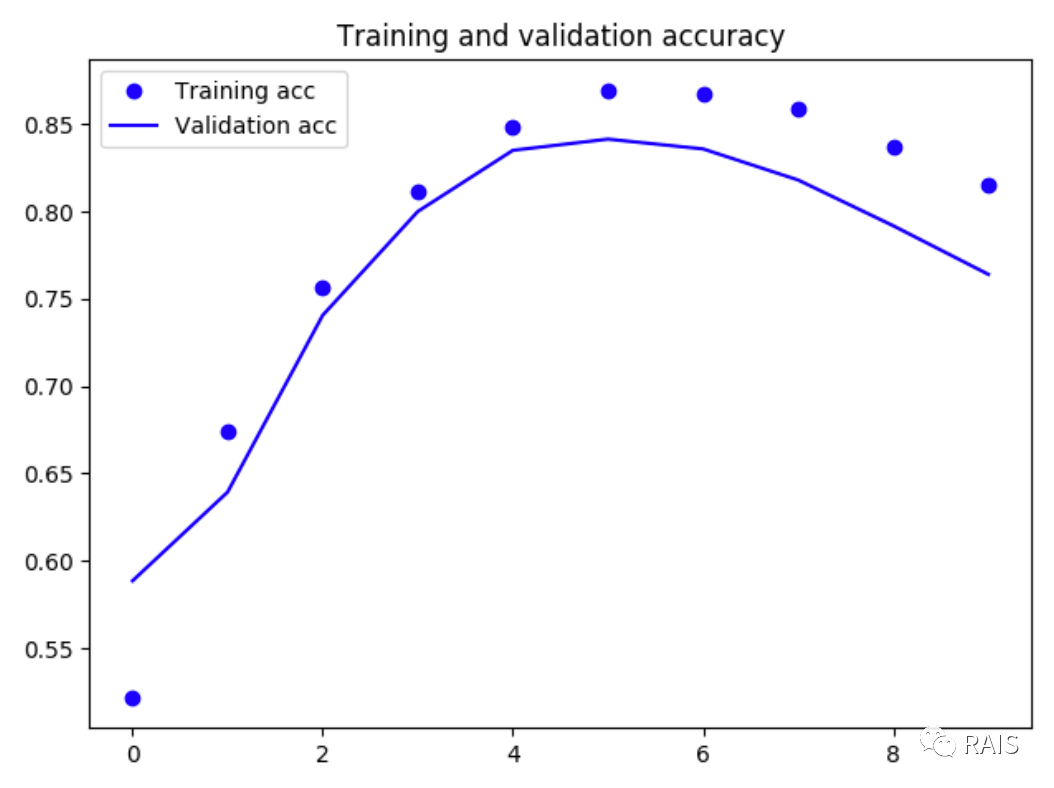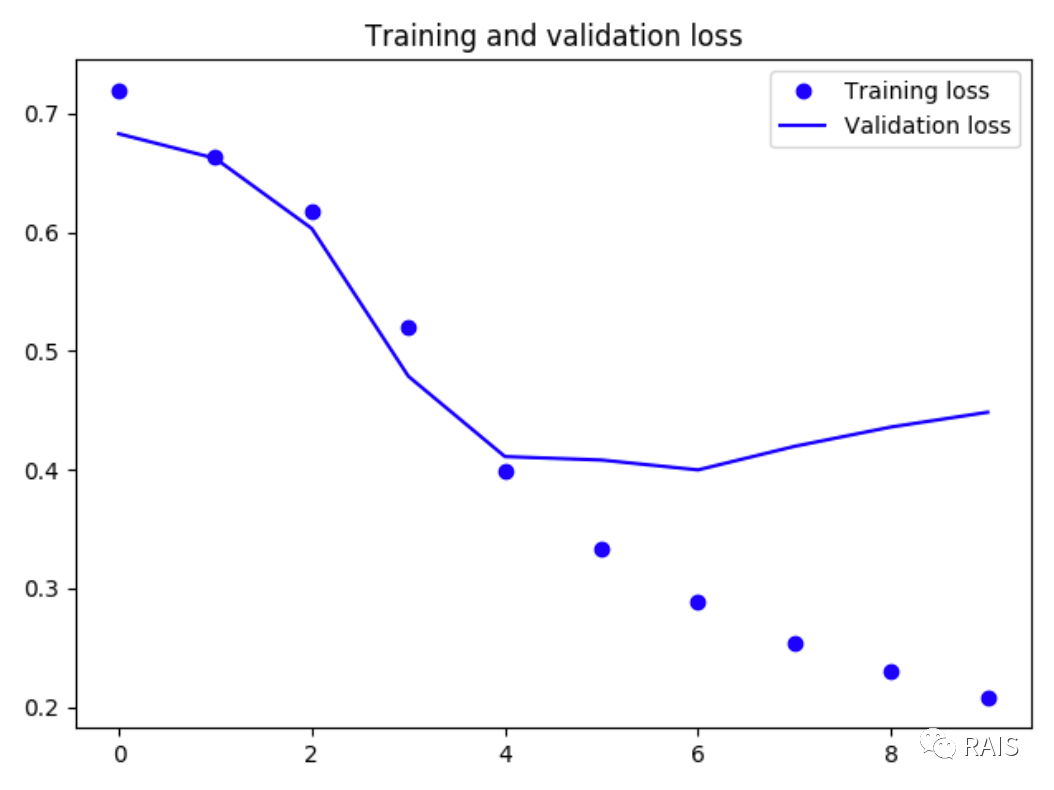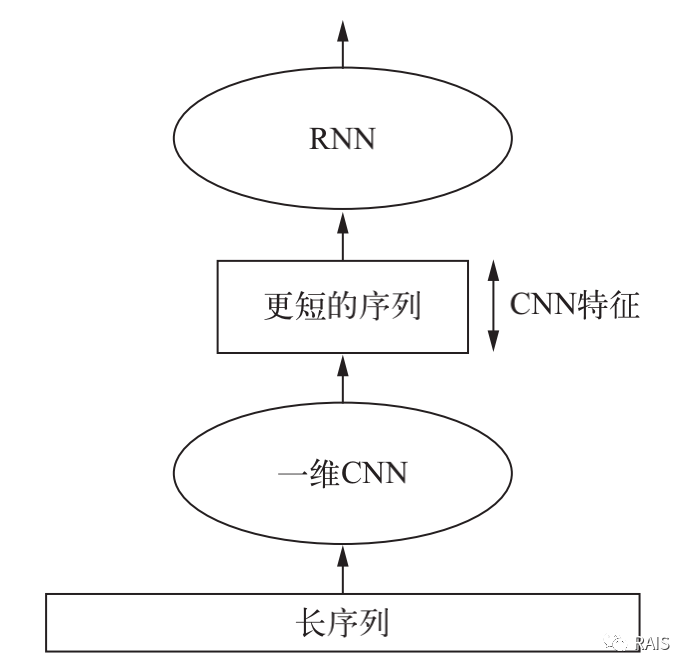# 卷积神经网络处理文本序列

1年前   阅读数 77 0``````#!/usr/bin/env python3
​
import time
​
import matplotlib.pyplot as plt
from keras import layers
from keras.datasets import imdb
from keras.models import Sequential
from keras.optimizers import RMSprop
from keras.preprocessing import sequence
​
​
def imdb_run():
max_features = 10000
max_len = 500
(x_train, y_train), (x_test, y_test) = imdb.load_data(num_words=max_features)
x_train = sequence.pad_sequences(x_train, maxlen=max_len)
x_test = sequence.pad_sequences(x_test, maxlen=max_len)
​
model = Sequential()
model.add(layers.Embedding(max_features, 128, input_length=max_len))
model.add(layers.Conv1D(32, 7, activation='relu'))
model.add(layers.MaxPooling1D(5))
model.add(layers.Conv1D(32, 7, activation='relu'))
model.add(layers.GlobalMaxPooling1D())
model.add(layers.Dense(1))
model.summary()
model.compile(optimizer=RMSprop(lr=1e-4), loss='binary_crossentropy', metrics=['acc'])
history = model.fit(x_train, y_train, epochs=10, batch_size=128, validation_split=0.2)
​
# 画图
acc = history.history['acc']
val_acc = history.history['val_acc']
loss = history.history['loss']
val_loss = history.history['val_loss']
epochs = range(1, len(loss) + 1)
plt.plot(epochs, acc, 'bo', label='Training acc')
plt.plot(epochs, val_acc, 'b', label='Validation acc')
plt.title('Training and validation accuracy')
plt.legend()
plt.show()
plt.figure()
plt.plot(epochs, loss, 'bo', label='Training loss')
plt.plot(epochs, val_loss, 'b', label='Validation loss')
plt.title('Training and validation loss')
plt.legend()
plt.show()
​
​
if __name__ == "__main__":
time_start = time.time()
imdb_run()
time_end = time.time()
print('Time Used: ', time_end - time_start)
````````````model = Sequential()
model.add(layers.Conv1D(32, 5, activation='relu',input_shape=(None, float_data.shape[-1])))
model.add(layers.MaxPooling1D(3))
​model.add(layers.Conv1D(32, 5, activation='relu'))
model.add(layers.GRU(32, dropout=0.1, recurrent_dropout=0.5))
model.add(layers.Dense(1))
``````

• 本文首发自微信公众号：RAIS

我有话说: